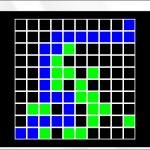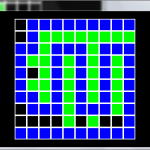# A* Pathfinding with Moai SDKIn preparation of the next gaming jam, I started evaluating Moai SDK, a LUA game framework which gained more widespread recognition last year, when Double Fine selected it to use be used for their Kickstarter adventure game. To get a good idea of a few different aspects of the SDK, I decided to try to make a pathfinding demo, specifically implementing the well-known, and equally well-documented A* algorithm. This tutorial assumes that you have a basic idea of Moai concepts – if that is not the case, I recommend their wiki page.

To get started, let’s create a Moai window, and add a layer to it we can use to render to:

```-- set up window
MOAISim.openWindow("Pathfinder", 640, 360)
viewport = MOAIViewport.new()
viewport:setScale(320, 180)
viewport:setSize(640, 360)

-- Create render stack
renderTable = {}
MOAIRenderMgr.setRenderTable(renderTable)

-- Create layer
layer = MOAILayer2D.new()
layer:setViewport(viewport)
table.insert(renderTable, layer)
```

To keep things simple, we’ll represent the world as a grid of empty and filled squares. Moai provides us with a class for this:

```-- set up collision map
local map = {}

-- Create tile grid
grid = MOAIGrid.new()
grid:initRectGrid(10, 10, 16, 16)
```

Next, we load up the tiles to display obstacles, and our path around them:

```-- Load sprite map
mapTiles = MOAITileDeck2D.new()
mapTiles:setTexture("map.png")
mapTiles:setSize(4,1)

-- Create a prop for tile grid
prop = MOAIProp2D.new()
prop:setDeck(mapTiles)
prop:setGrid(grid)
prop:setLoc(-80, -80)
layer:insertProp(prop)
```

In order to keep the MOAIGrid in sync with our collision map, we need a couple of helper functions:

```function resetGrid()
-- reset collision map
for i = 1, 100 do
map[i] = false
end
updateGrid()
end

function updateGrid()
local x = 1
local y = 1
-- set each grid tile according to the collision map
for i = 1, #map do
local val = 4
if map[i] then
val = 2
end

grid:setTile(x, y, val)

x = x + 1
if x > 10 then
x = 1
y = y + 1
end
end
end
```

We will allow the user to set tiles as empty / blocked by clicking on them:

```MOAIInputMgr.device.mouseLeft:setCallback(
function(down)
-- only handle mouse down even
if not down then
return
end

-- convert mouse position to tile position
x,y = layer:wndToWorld(MOAIInputMgr.device.pointer:getLoc())
x = math.floor((x + 80) / 16) + 1
y = math.floor((y + 80) / 16) + 1

if x < 1 or x > 10 or y < 1 or y > 10 then
return
end

-- update collision map
local idx = (y - 1) * 10 + x
map[idx] = not map[idx]
updateGrid()
end
)
```

Additionally, the user will be able to reset the map using the ‘R’ key, and to search for a path using ‘Space’:

```MOAIInputMgr.device.keyboard:setCallback (
function(key, down)
-- only handle key down event
if not down then
return
end

if key == 32 then
findPath()
elseif key == 114 then
reset()
end
end
)

function findPath()
local finder = Pathfinder:new()
finder:setMap(map, 10)
local path = finder:findPath({x=1, y=1}, {x=10,y=10})
local i = path
while i do
grid:setTile(i.x, i.y, 3)
i = i.next
end
end
```This concludes the MOAI-specific parts of this tutorial. For a great introduction to A*, and boat-loads of other pathfinding algorithms, please see Amit’s A* Pages. My own implementation of A* in LUA can be found under pathfinder.lua. The demo code and tile map are also available.